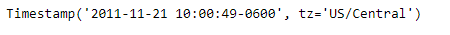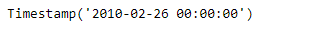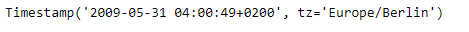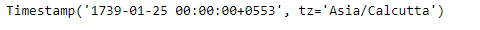# Python | Pandas Timestamp.fromordinal

• Last Updated : 14 Jan, 2019

Python is a great language for doing data analysis, primarily because of the fantastic ecosystem of data-centric python packages. Pandas is one of those packages and makes importing and analyzing data much easier.

Pandas` Timestamp.fromordinal()` function return a Timestamp object when passed an ordinal, the function translate and convert it to a ts object. By definition there cannot be any tz info on the ordinal itself.

Attention geek! Strengthen your foundations with the Python Programming Foundation Course and learn the basics.

To begin with, your interview preparations Enhance your Data Structures concepts with the Python DS Course. And to begin with your Machine Learning Journey, join the Machine Learning - Basic Level Course

Syntax :Timestamp.fromordinal()

Parameters :

ordinal : date corresponding to a proleptic Gregorian ordinal

freq : Offset which Timestamp will have

tz : Time zone for time which Timestamp will have

Return : Timestamp

Example #1: Use `Timestamp.fromordinal()` function to translate the given Timestamp object based on the passed ordinal value.

 `# importing pandas as pd``import` `pandas as pd`` ` `# Create the Timestamp object``ts ``=` `pd.Timestamp(year ``=` `2011``,  month ``=` `11``, day ``=` `21``,``                  ``hour ``=` `10``, second ``=` `49``, tz ``=` `'US/Central'``)`` ` `# Print the Timestamp object``print``(ts)`

Output :Now we will use the `Timestamp.fromordinal()` function to convert the given Timestamp object.

 `# convert the given Timestamp object``# based on the passed ordinal value``ts.fromordinal(ordinal ``=` `733829``)`

Output :As we can see in the output, the `Timestamp.fromordinal()` function has returned a new Timestamp object based on the passed ordinal value.

Example #2: Use `Timestamp.fromordinal()` function to translate the given Timestamp object based on the passed ordinal value. Also set the timezone.

 `# importing pandas as pd``import` `pandas as pd`` ` `# Create the Timestamp object``ts ``=` `pd.Timestamp(year ``=` `2009``, month ``=` `5``, day ``=` `31``,``                  ``hour ``=` `4``, second ``=` `49``, tz ``=` `'Europe/Berlin'``)`` ` `# Print the Timestamp object``print``(ts)`

Output :Now we will use the `Timestamp.fromordinal()` function to convert the given Timestamp object.

 `# convert the given Timestamp object``# based on the passed ordinal value``ts.fromordinal(ordinal ``=` `634816``, tz ``=` `'Asia / Calcutta'``)`

Output :As we can see in the output, the `Timestamp.fromordinal()` function has returned a new Timestamp object based on the passed ordinal value. The function has also set the timezone for the returned Timestamp object.

My Personal Notes arrow_drop_up